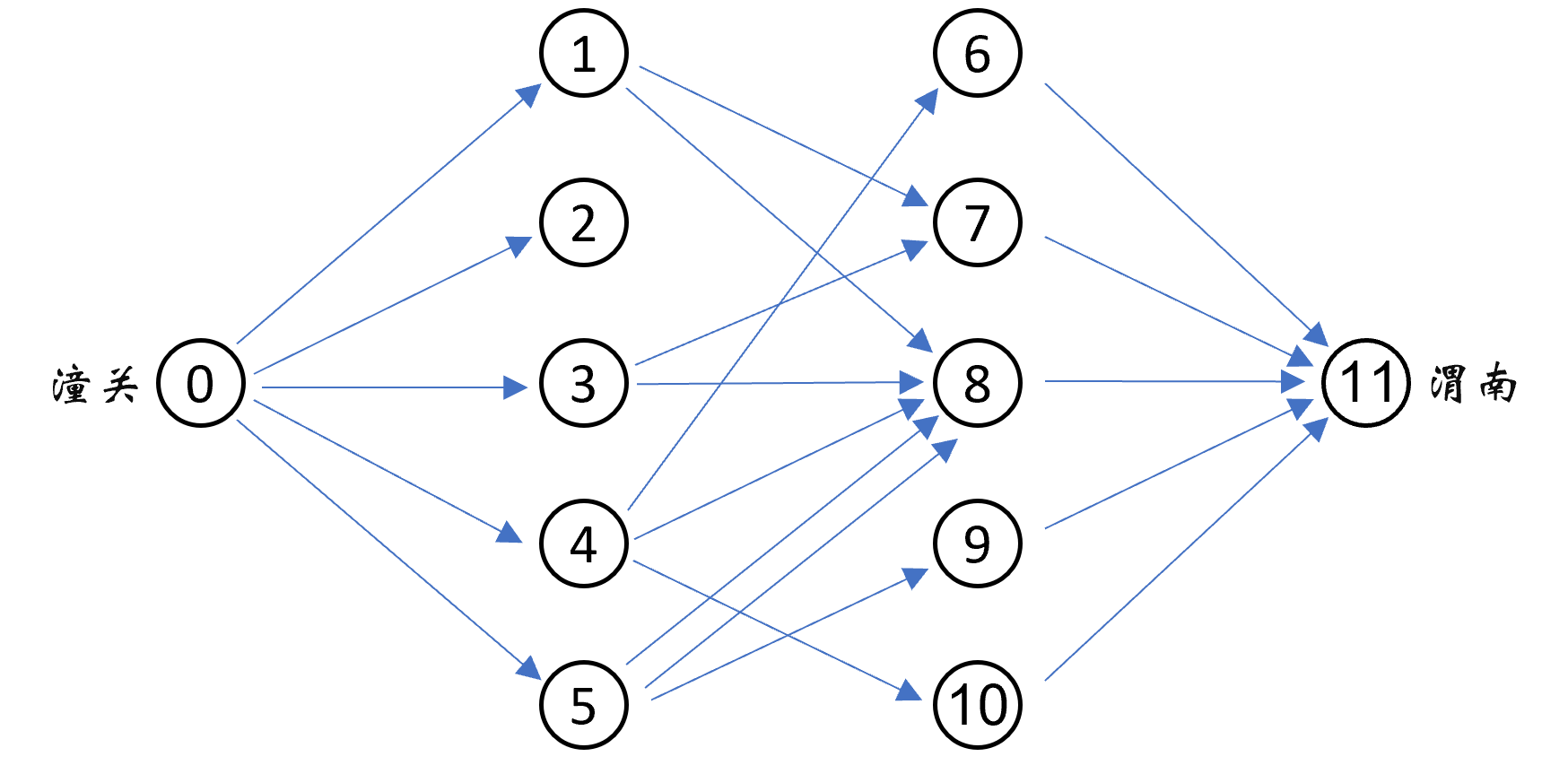# #704. 马超战潼关

1. 潼关附近的地图可以近似看成一个 $2n+2$ 个点的有向无环图。其中 $0$ 号点为潼关， $2n+1$ 号点为渭南。
2. $0$ 号点有到 $1,2,\cdots,n$ 号点的有向边，共计 $n$ 条。
3. $n+1,n+2,\cdots,2n$ 号点有连向 $2n+1$ 的有向边，共计 $n$ 条。
4. 除此之外还有 $m$ 条边，第 $i$ 条边为从 $u_i$ 到 $n+v_i$ 的边。其中 $1 \le u_i, v_i \le n$。
5. 不保证图中无重边。

### 样例一

#### input

5 10
5 3
4 1
1 3
3 3
1 2
4 5
5 3
4 3
5 4
3 2



#### output

4



#### explanation1. $(0, 1), (0, 3), (0, 4), (0, 5)$；
2. $(0, 4), (0, 5), (7, 11), (8, 11)$；
3. $(0, 4), (5, 9), (7, 11), (8, 11)$；
4. $(0, 4), (7, 11), (8, 11), (9, 11)$。

### 数据范围

$1$ $2n+m\leq 20$ $5$
$2$ $n\leq 10$ $10$
$3$ $n\leq 16$ $20$
$4$ $n\leq 20$ $10$
$5$ $m$ 为偶数，$u_{2i-1}=u_{2i},v_{2i-1}=v_{2i}$（$1\leq i\leq \frac{m}{2}$） $20$
$6$ $n\leq 32$ $15$
$7$ $n\leq 46$ $20$